• matplotlib柱状图宽度
千次阅读
2022-02-18 15:03:29

# Matplotlib柱状图（代码+注释详解）

柱状图是一种用矩形柱来表示数据分类的图表，柱状图可以垂直绘制，也可以水平绘制，它的高度与其所表示的数值成正比关系。柱状图显示了不同类别之间的比较关系，图表的水平轴 X 指定被比较的类别，垂直轴 Y 则表示具体的类别值。

Matplotlib 提供了bar()函数来绘制柱状图，它可以应用在 MATLAB 样式以及面向对象的绘图方法中。当它与 axes 对象一起使用时，其语法格式如下：

ax.bar(x, height, width, bottom, align)


该函数的参数说明，如下表所示：

bar()函数参数说明

x 一个标量序列，代表柱状图的x坐标，默认x取值是每个柱状图所在的中点位置，或者也可以是柱状图左侧边缘位置。

height 一个标量或者是标量序列，代表柱状图的高度。

width 可选参数，标量或类数组，柱状图的默认宽度值为 0.8。

bottom 可选参数，标量或类数组，柱状图的y坐标默认为None。

algin 有两个可选项 {“center”,“edge”}，默认为 ‘center’，该参数决定 x 值位于柱状图的位置。

该函数的返回值是一个 Matplotlib 容器对象，该对象包含了所有柱状图。

下面是一个关于 Matplotlib 柱状图的简单示例。它用来显示了不同编程语言的学习人数。

import matplotlib.pyplot as plt
#创建图形对象
fig = plt.figure()
#添加子图区域，参数值表示[left, bottom, width, height ]
#准备数据
langs = ['C', 'C++', 'Java', 'Python', 'PHP']
students = [23,17,35,29,12]
#绘制柱状图
ax.bar(langs,students)
plt.show()


输出结果如下：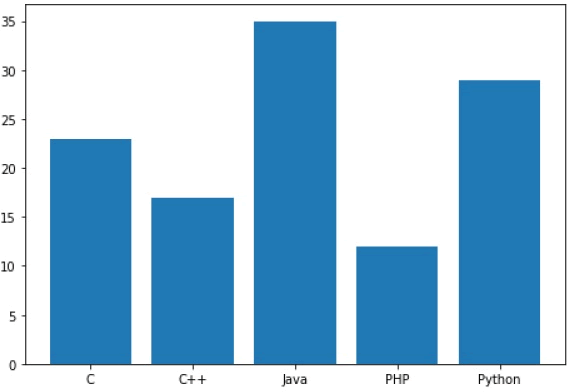图1：matplotlib bar()绘图

通过调整柱状图的宽度，可以实现在同一 x 轴位置绘制多个柱状图。您可以将它们设置成不同的颜色，从而使它们更容易区分。下面示例描述了某工程学院过去四年中，三个专业录取的统招学生数量。

import numpy as np
import matplotlib.pyplot as plt
#准备数据
data =
[[30, 25, 50, 20],
[40, 23, 51, 17],
[35, 22, 45, 19]]
X = np.arange(4)
fig = plt.figure()
#添加子图区域
#绘制柱状图
ax.bar(X + 0.00, data, color = 'b', width = 0.25)
ax.bar(X + 0.25, data, color = 'g', width = 0.25)
ax.bar(X + 0.50, data, color = 'r', width = 0.25)


上述代码执行后，将显示四个柱状图，将每个柱状图又均分为三个小柱状图，每个柱状图占据 0.25 个单位。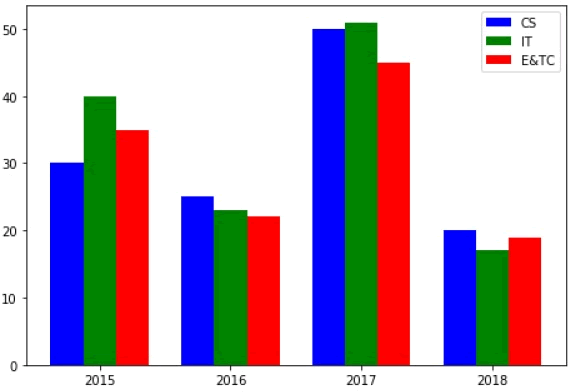图2：matplotlib绘图

柱状图除了上述使用方法外，还有另外一种堆叠柱状图。所谓堆叠柱状图就是将不同数组别的柱状图堆叠在一起，堆叠后的柱状图高度显示了两者相加的结果值。

bar() 函数提供了一个可选参数bottom，该参数可以指定柱状图开始堆叠的起始值，一般从底部柱状图的最大值开始，依次类推。

下面是一个不同国家参加奥林匹克运动会所得奖牌（金银铜）的柱状堆叠图示例，如下所示：

import numpy as np
import matplotlib.pyplot as plt
countries = ['USA', 'India', 'China', 'Russia', 'Germany']
bronzes = np.array([38, 17, 26, 19, 15])
silvers = np.array([37, 23, 18, 18, 10])
golds = np.array([46, 27, 26, 19, 17])
# 此处的 _ 下划线表示将循环取到的值放弃，只得到[0,1,2,3,4]
ind = [x for x, _ in enumerate(countries)]
#绘制堆叠图
plt.bar(ind, golds, width=0.5, label='golds', color='gold', bottom=silvers+bronzes)
plt.bar(ind, silvers, width=0.5, label='silvers', color='silver', bottom=bronzes)
plt.bar(ind, bronzes, width=0.5, label='bronzes', color='#CD853F')
#设置坐标轴
plt.xticks(ind, countries)
plt.ylabel("Medals")
plt.xlabel("Countries")
plt.legend(loc="upper right")
plt.title("2019 Olympics Top Scorers")
plt.show()


在上述代码中，第一次调用plt.bar()绘制了黄色柱状图， 第二次调用plot.bar()时绘制了灰色柱状图，最后一次调用plt.bar()则绘制最底部的柱状图。两个柱状图相接触的位置就是顶部与底部的位置，这样就构成了柱状堆叠图。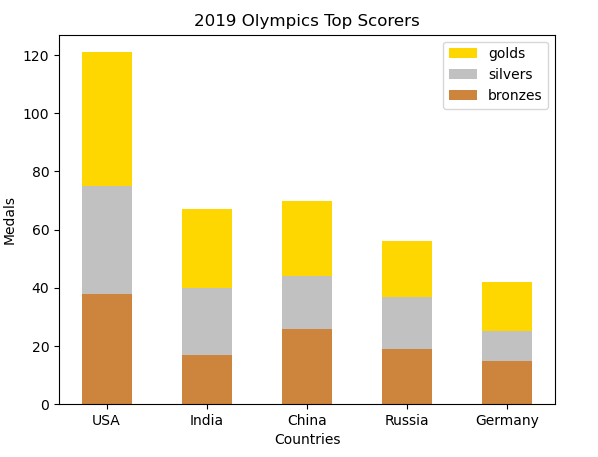图3：柱状堆叠图matlab Matplotlib python
更多相关内容
• matplotlib是Python中最基本的绘图库，而柱状图又是使用频率较高的可视化图形。下面我就在有pyecharts库和R语言，echarts绘图经验的基础上，简单得摸索一下用matplotlib绘制柱状图的方法。代码如下：# 导入相关的库...

matplotlib是Python中最基本的绘图库，而柱状图又是使用频率较高的可视化图形。下面我就在有pyecharts库和R语言，echarts绘图经验的基础上，简单得摸索一下用matplotlib绘制柱状图的方法。代码如下：

# 导入相关的库

import pandas as pd

import numpy as np

import matplotlib.pyplot as plt

# 在线显示

%matplotlib inline

# 定义画布的大小

fig = plt.figure(figsize = (15,8))

# 添加主标题

plt.title("各品牌汽车的销量")

# 设置X周与Y周的标题

# plt.xlabel("品牌")

# plt.ylabel("销量")

# 显示网格线

# plt.grid(True)

# 设置 x轴 数据

x = np.array(["宝马","奔驰","奥迪","马自达","大众","布加迪","兰博基尼","法拉利","本田","丰田"])

# 设置 y轴 数据

y = np.array([1000,800,600,400,300,250,150,100,80,50])

# 直接绘制简单的柱状图

# plt.bar(x,y)

# 绘制柱状图，并把每根柱子的颜色设置为洋红色

# plt.bar(x,y,color = "m")

# 绘制柱状图，并把每根柱子的颜色设置为洋红色，顺便设置每根柱子的宽度

# plt.bar(x,y,color = "m",width = 0.6)

# 绘制柱状图，并给每根柱子的颜色根据自己的喜好来自定义

plt.bar(x,y,color = ["red","yellow","green","blue","black","gold","pink","purple","violet","Chocolate"])

# 保存输出绘制的图形到指定的路径中

plt.savefig(r"C:\Users\QDM\Desktop\car.png")

运行结果①：

# 直接绘制简单的柱状图

plt.bar(x,y)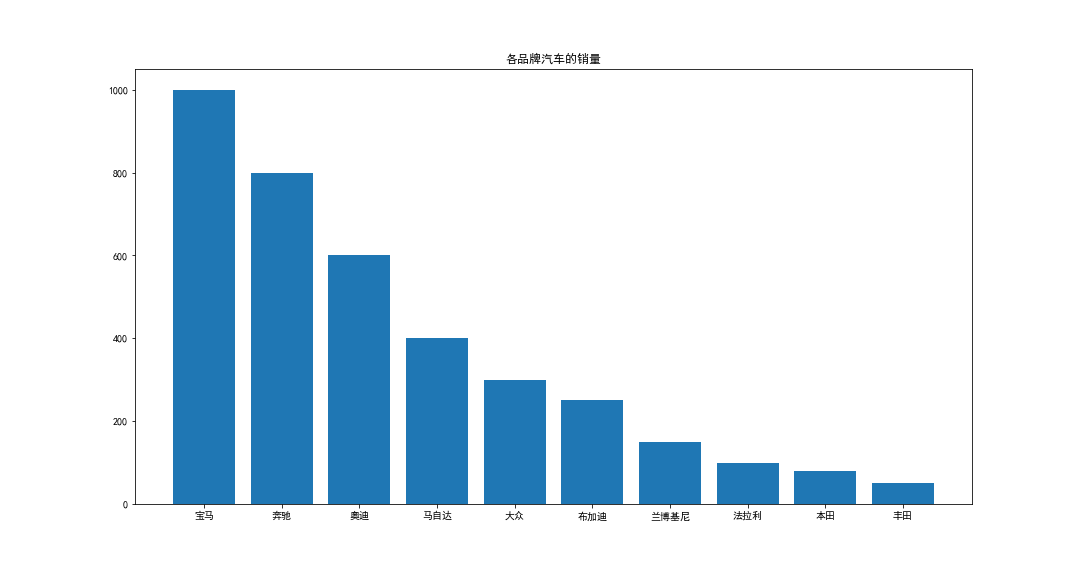运行结果②：

# 绘制柱状图，并把每根柱子的颜色设置为洋红色

plt.bar(x,y,color = "m")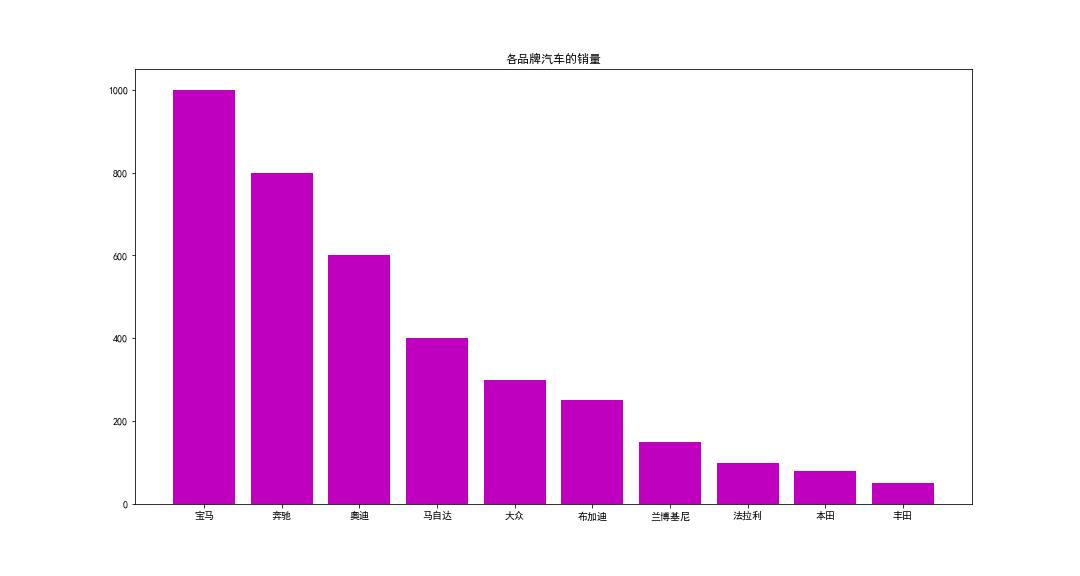运行结果③：

# 绘制柱状图，并把每根柱子的颜色设置为洋红色，顺便设置每根柱子的宽度

plt.bar(x,y,color = "m",width = 0.6)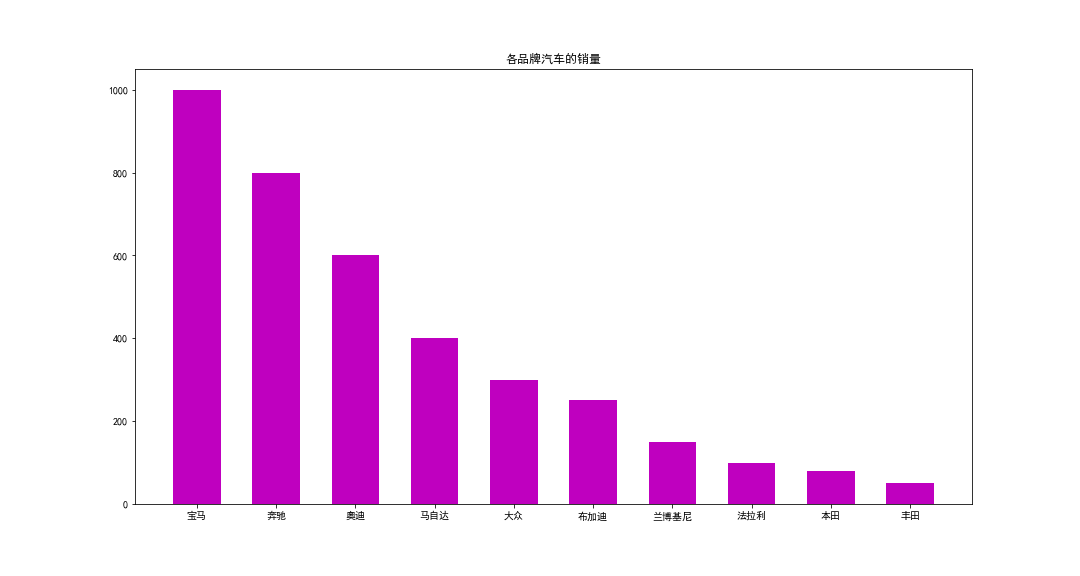运行结果④：

# 绘制柱状图，并给每根柱子的颜色根据自己的喜好来自定义

plt.bar(x,y,color = ["red","yellow","green","blue","black","gold","pink","purple","violet","Chocolate"])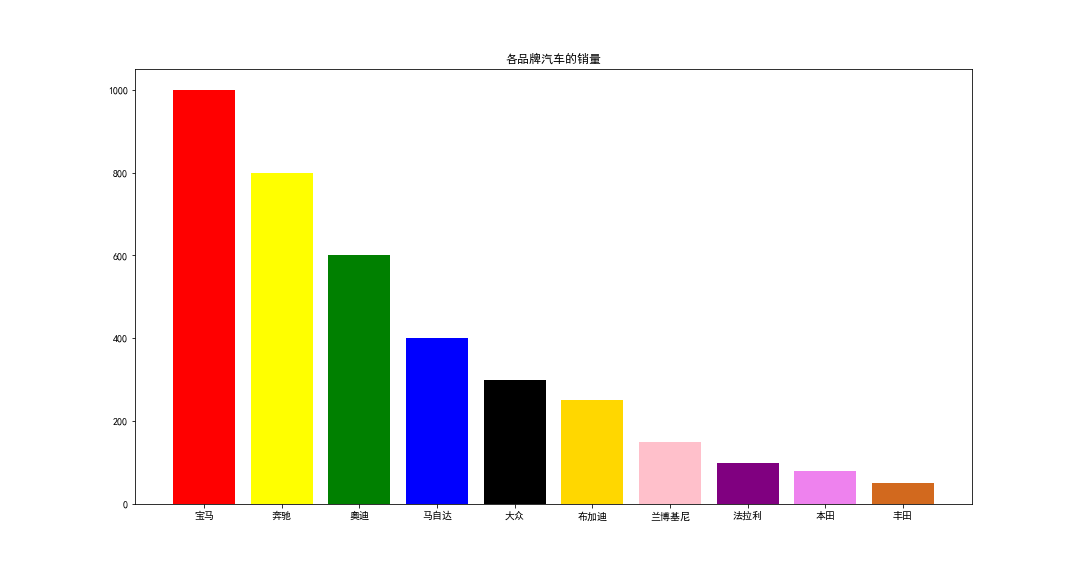另外，我们一般将纵向的称为“柱型图”，而横向的成为“柱状图”。两者的共同点是用于相同实物的量之对比，不同点是柱型图一般用于表示较少对象之间的对比，但如果需要对比的条目较多的话，还用柱型图就会使画面有一种拥挤不堪的感觉。这时，我们就需要用到另一种“柱型图”——柱状图（横向）。

其实在matplotlib中，要绘制柱形图也非常简单，只需把bar()参数改为barh()即可，如下：

# 导入相关的库

import pandas as pd

import numpy as np

import matplotlib.pyplot as plt

# 在线显示

%matplotlib inline

# 定义画布的大小

fig = plt.figure(figsize = (15,8))

# 添加主标题

plt.title("各品牌汽车的销量")

# 设置X周与Y周的标题

# plt.xlabel("品牌")

# plt.ylabel("销量")

# 显示网格线

# plt.grid(True)

# 设置 x轴 数据

x = np.array(["丰田","本田","法拉利","兰博基尼","布加迪","大众","马自达","奥迪","奔驰","宝马"])

# 设置 y轴 数据

# 值得注意的是，参数的排列最好是排一个升序或降序，这样画出来的图形会因为比较有规律感，这样会显得比较好看一点

y = np.array([50,80,100,150,250,300,400,600,800,1000])

# 直接绘制简单的柱状图

# plt.barh(x,y)

# 绘制柱状图，并把每根柱子的颜色设置为洋红色

# plt.barh(x,y,color = "m")

# 绘制柱状图，并把每根柱子的颜色设置为洋红色，顺便设置每根柱子的宽度

# plt.barh(x,y,color = "m",width = 0.6)

# 绘制柱状图，并给每根柱子的颜色根据自己的喜好来自定义

plt.barh(x,y,color = ["red","yellow","green","blue","black","gold","pink","purple","violet","Chocolate"])

# 保存输出绘制的图形到指定的路径中

plt.savefig(r"C:\Users\QDM\Desktop\car.png")

运行结果：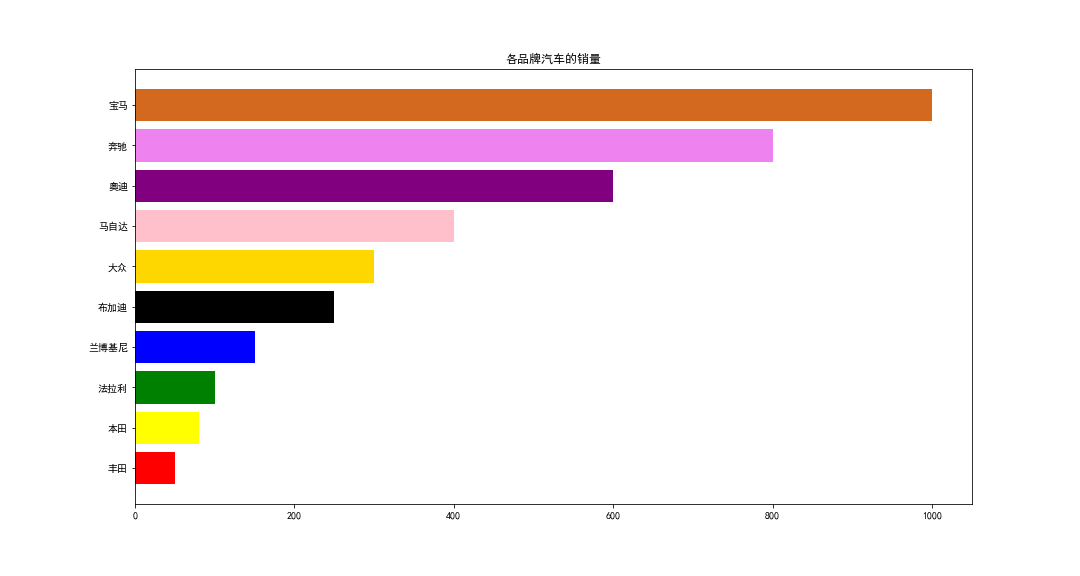展开全文• 决定了柱子的宽度，仅代表形状宽度而已 决定了柱子距离x轴的高度，默认为None，即表示与x轴距离为0 事实上，，，，这四个参数确定了柱体的位置和大小。默认情况下，left为柱体的居中位置（可以通过align参数来改变...

Base python matlibplot库——

# 一、主要参数介绍：

bar(left, height, width=0.8, bottom=None, **kwargs)

1. left为和分类数量一致的数值序列，序列里的数值数量决定了柱子的个数，数值大小决定了距离0点的位置
2. height为分类变量的数值大小，决定了柱子的高度
3. width决定了柱子的宽度，仅代表形状宽度而已
4. bottom决定了柱子距离x轴的高度，默认为None，即表示与x轴距离为0

事实上，leftheightwidthbottom这四个参数确定了柱体的位置和大小。默认情况下，left为柱体的居中位置（可以通过align参数来改变left值的含义）

例程：（以下操作都是按照这个数据）

# 正常显示中文标签
plt.rcParams['font.sans-serif']=['SimHei']
# 用来正常显示负号
plt.rcParams['axes.unicode_minus']=False

plt.rcParams['axes.labelsize']=16
plt.rcParams['xtick.labelsize']=14
plt.rcParams['ytick.labelsize']=14
plt.rcParams['legend.fontsize']=12
plt.rcParams['figure.figsize']=[16,6]
# 使用样式
plt.style.use("ggplot")
label = ["a","b","c","d","e"]
x = [0,1,2,3,4]
y = [30,20,15,25,10]


# 二、柱子宽度设置

width决定了柱子的宽度，仅代表形状宽度而已，默认为0.8

fig = plt.figure()

# 生成第一个子图在1行2列第一列位置

# 生成第二子图在1行2列第二列位置

# 绘图并设置柱子宽度0.5
ax1.bar(x, y, width=0.5)

# 绘图默认柱子宽度0.8
ax2.bar(x, y)
plt.show()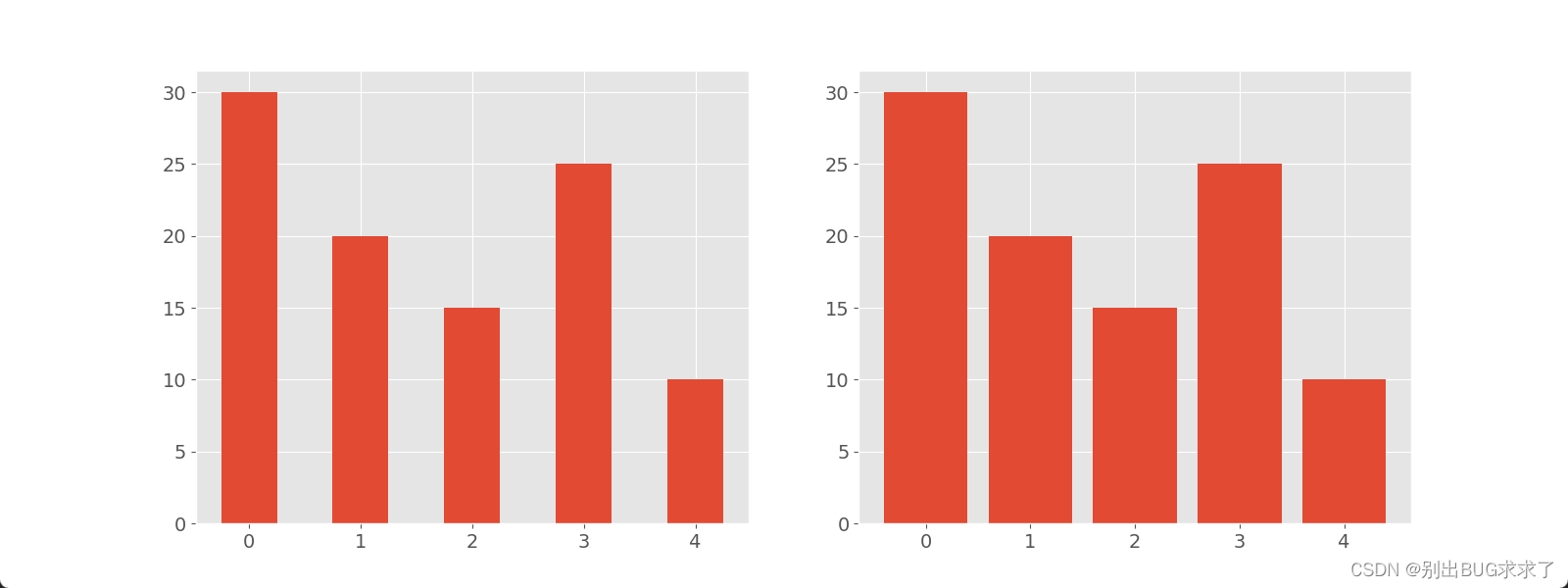# 三、设置X轴刻度用label显示

设置x轴刻度（tick_label）用 label = [“a”,“b”,“c”,“d”,“e”] 显示

fig = plt.figure()

# 生成第一个子图在1行2列第一列位置

# 生成第二子图在1行2列第二列位置

# 绘图并设置柱子宽度0.5
ax1.bar(x, y, width=0.5)

# 绘图默认柱子宽度0.8,设置x轴刻度（tick_label）用 label = ["a","b","c","d","e"] 显示
ax2.bar(x, y,tick_label=label)
plt.show()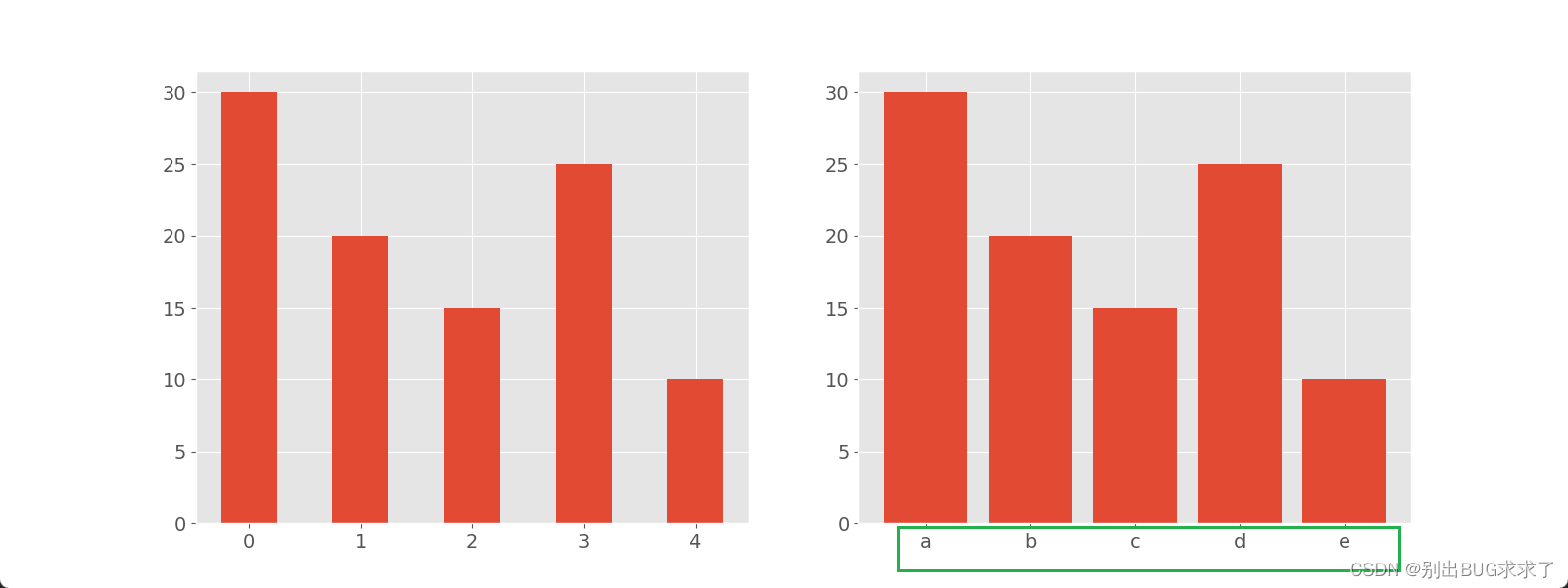# 四、设置柱子距离x轴的高度

bottom决定了柱子距离x轴的高度，默认为None，即表示与x轴距离为0

fig,ax = plt.subplots(figsize=(8,5),dpi=80)

# 设置第一个柱子离x轴 为5 ，第二、三、五个柱子不变，第四个柱子离x轴 为8
ax.bar(x,y,width=0.3,bottom=[5,0,0,8,0])

plt.show()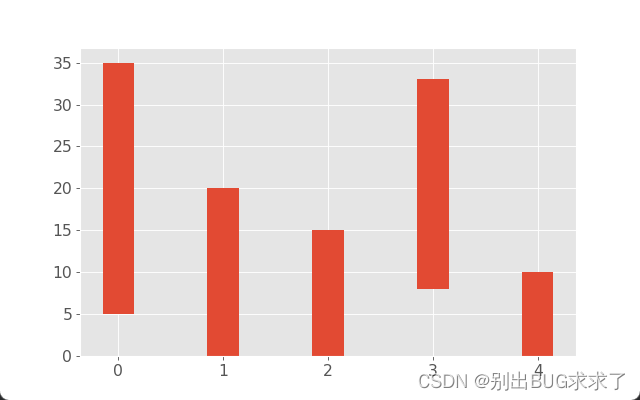# 五、设置柱体颜色

通过 facecolor(或fc) 关键字参数可以设置柱体颜色

通过 color 关键字参数 可以一次性设置多个颜色

fig = plt.figure()

# 生成第一个子图在1行2列第一列位置

# 生成第二子图在1行2列第二列位置

ax1.bar(x, y, fc='c')

ax2.bar(x, y,color=['r', 'g', 'b']) # 或者color='rgb' , color='#FFE4C4'

plt.show()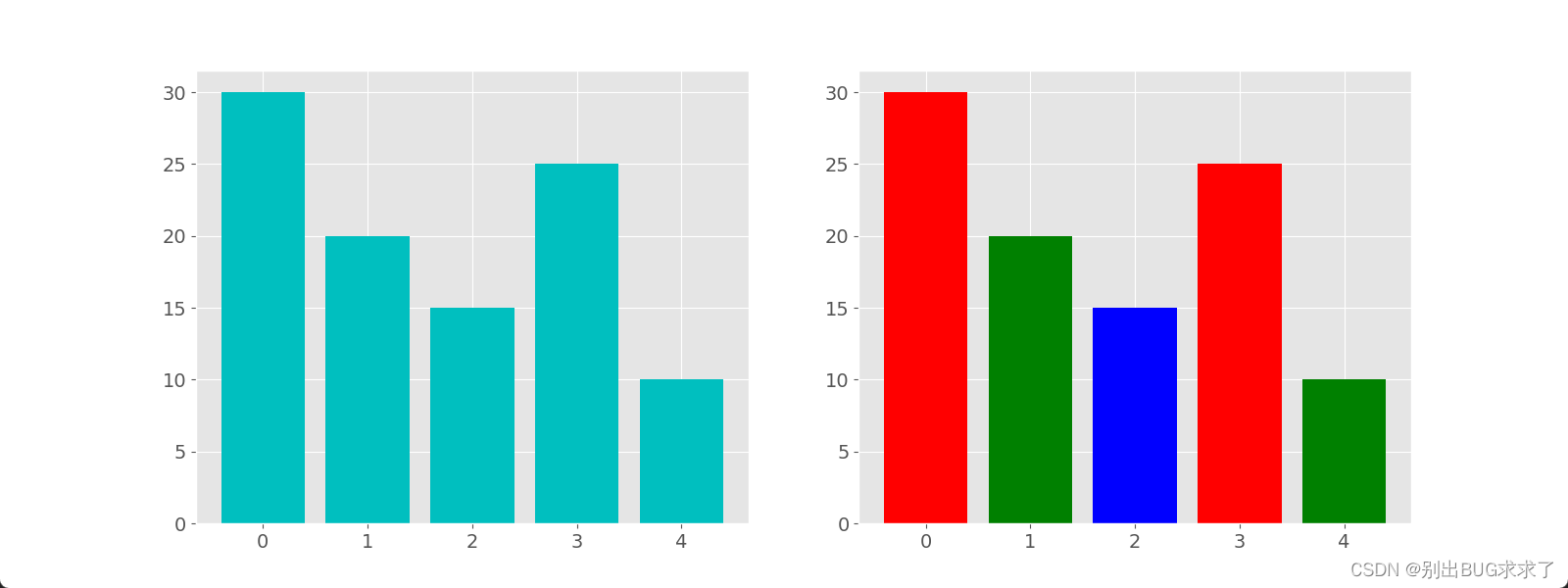# 六、设置柱体描边

相关的关键字参数为：

• edgecolor 或 ec 描边颜色
• linestyle 或 ls 描边样式
• linewidth 或 lw 描边宽度
# edgecolor 或 ec 描边颜色为（cyan），linestyle 或 ls 描边样式 （-.）
# linewidth 或 lw 描边宽度（5），柱子颜色（#EECFA1）
plt.bar(x, y, ec='c', ls='-.', lw=5,color='#EECFA1')
plt.show()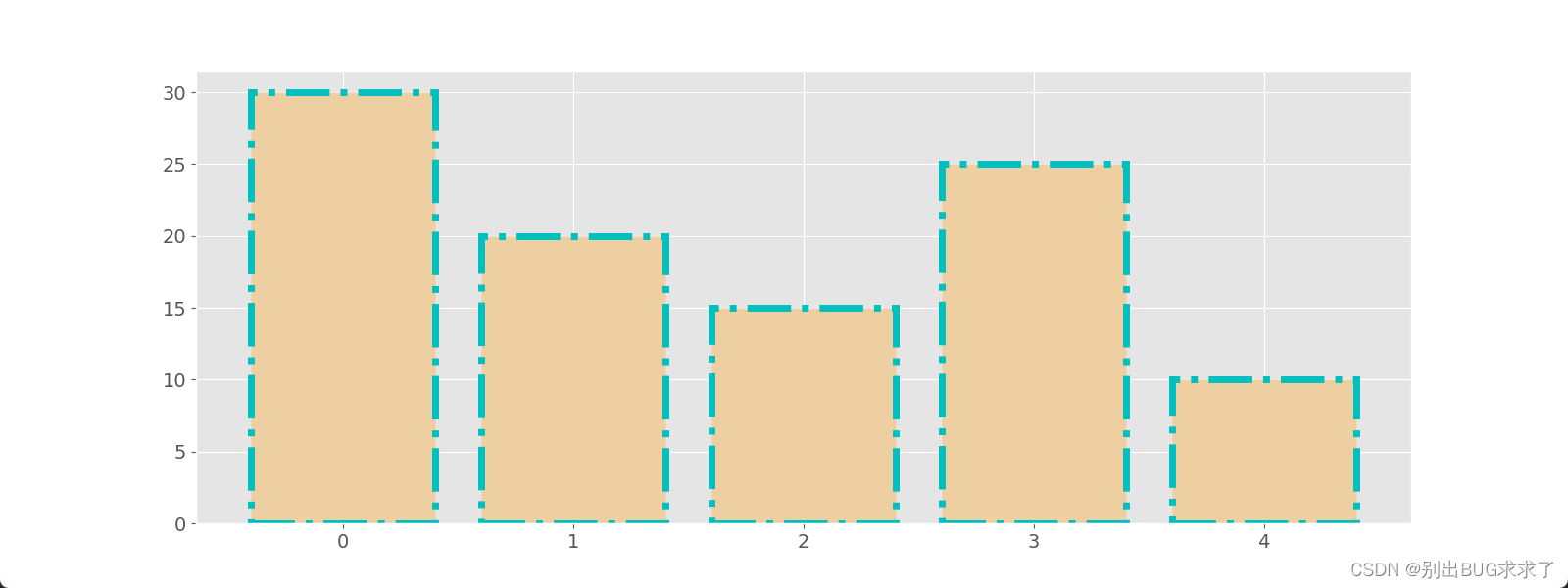# 七、设置柱体描边填充

hatch 关键字可用来设置填充样式，可取值为： / , \ , | , - , + , x , o , O , . , * 。

plt.bar(x, y, ec='c', ls='-.', lw=5,color='#EEDC82',hatch='*')
plt.show()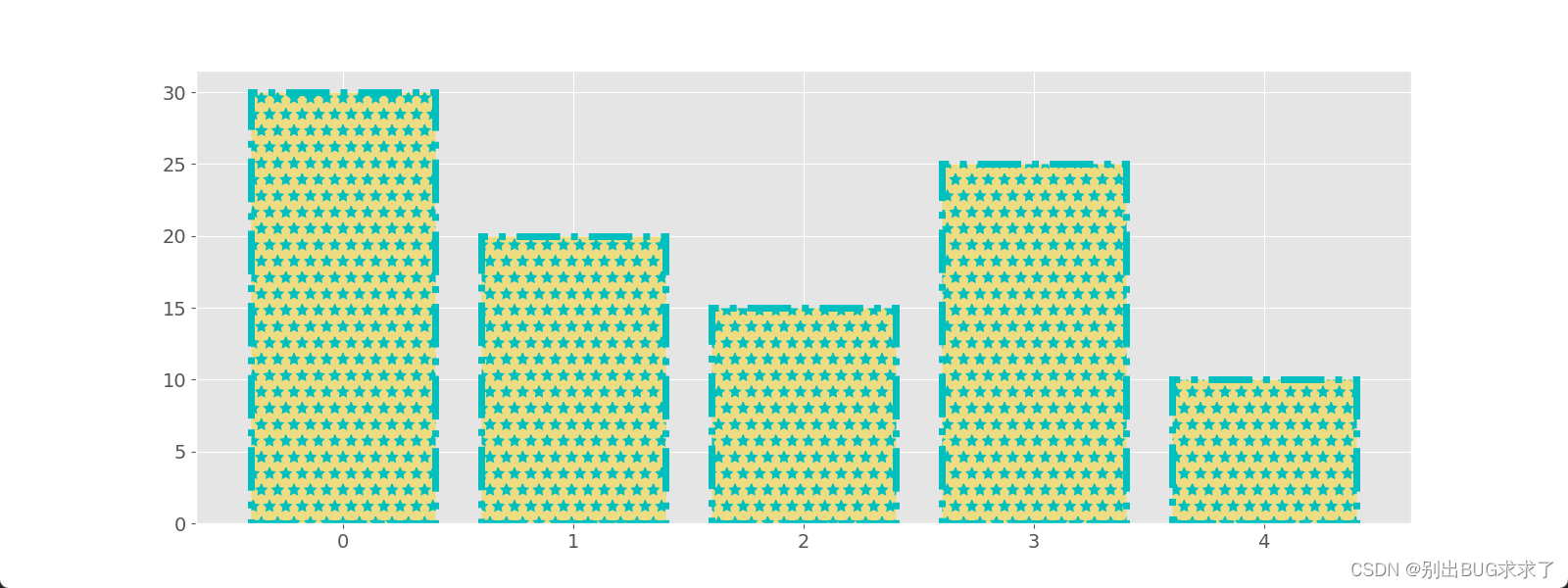# 八、不同柱状图类型绘制

## 1. 堆积柱状图

通过 bottom 参数，可以绘制堆叠柱状图。

#显示中文字体为SimHei
plt.rcParams["font.sans-serif"]=["SimHei"]

sale8 = [10,20,30,15,18]
sale9 = [10,12,24,32,8]

# x轴的刻度为1-5号衣服
labels = ["{}号衣服".format(i) for i in range(1,6)]

fig,ax = plt.subplots(figsize=(12,6),dpi=80)

ax.bar(range(len(sale8)),sale8,tick_label=labels,label="8月")

# 九月的bottom是sale8，也就是八月，所以九月在上边
ax.bar(range(len(sale9)),sale9,bottom=sale8,tick_label=labels,label="9月")
ax.legend()
plt.show()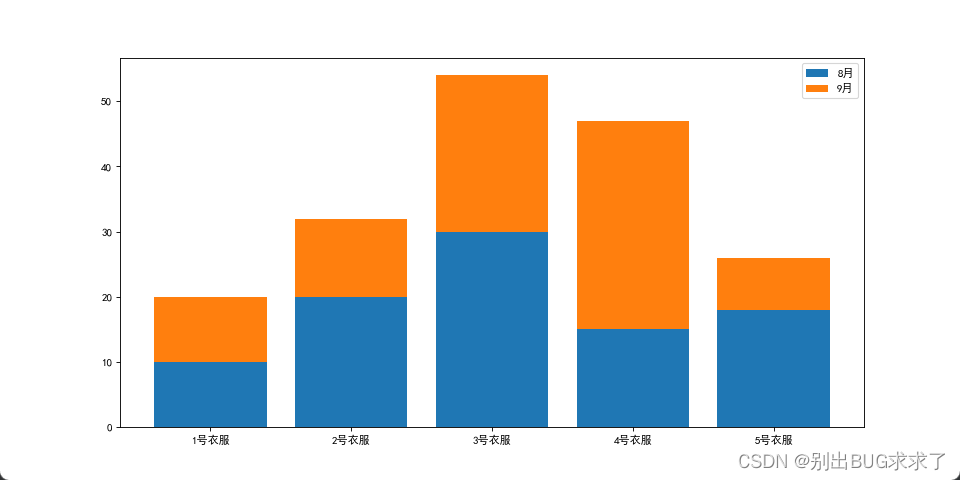## 2. 并列柱状图

绘制并列柱状图与堆叠柱状图类似，都是绘制多组柱体，只需要控制好每组柱体的位置和大小即可。

import numpy as np
#显示中文字体为SimHei
plt.rcParams['font.sans-serif']=['SimHei']

sale8 = [5,20,15,25,10]
sale9 = [10,15,25,30,5]

# x轴的刻度为1-5号衣服
labels = ["{}号衣服".format(i) for i in range(1,6)]

fig,ax = plt.subplots(figsize=(8,5),dpi=80)
width_1 = 0.4

ax.bar(np.arange(len(sale8)),sale8,width=width_1,tick_label=labels,label = "8月")

ax.bar(np.arange(len(sale9))+width_1,sale9,width=width_1,tick_label=labels,label="9月")

ax.legend()
plt.show()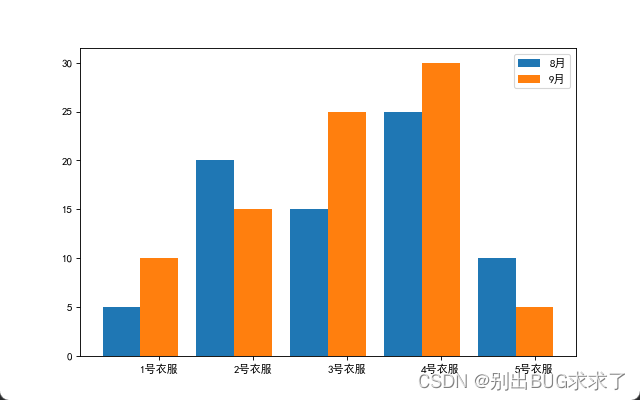## 3. 横状条形图

使用 barh 方法绘制条形图，plt.barh 方法的签名为：

barh(bottom, width, height=0.8, left=None, **kwargs)


可以看到与 plt.bar 方法类似。所以堆积条形图和并列条形图的画法与前面类似。

plt.barh(x, y)
plt.show()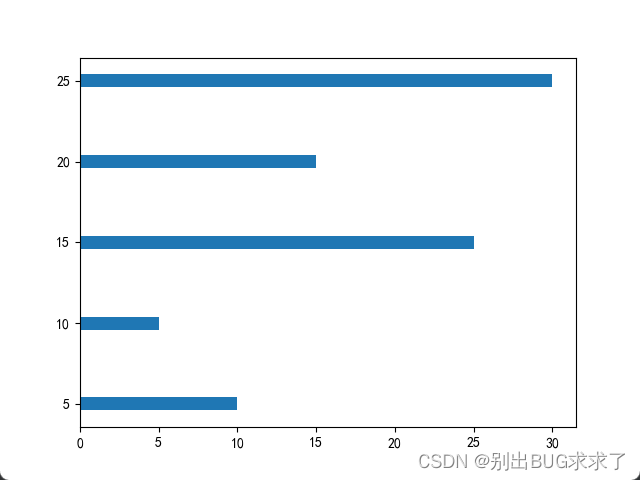## 4. 正负条形图

import numpy as np
import matplotlib.pyplot as plt

x = np.array([5, 20, 15, 25, 10])
y = np.array([11, 15, 22, 14, 5])

plt.barh(range(len(x)), x)
plt.barh(range(len(y)), -y)
plt.show()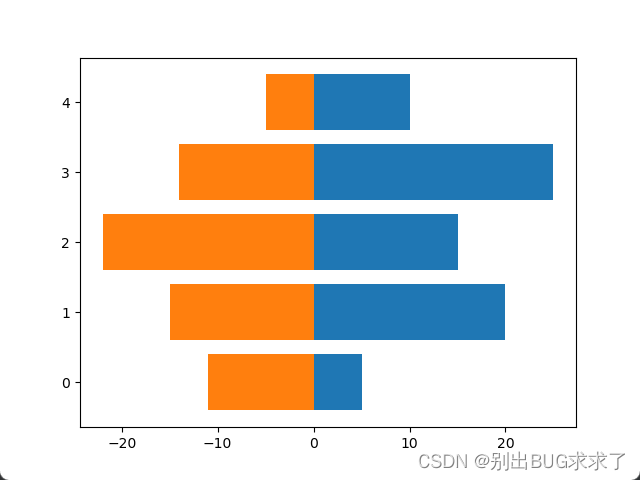# 九、案例介绍

## 1. 案例一：同一本书不同平台最低价比较

很多人在买一本书的时候，都比较喜欢货比三家，例如《python数据分析实战》在亚马逊、当当网、中国图书网、京东和天猫的最低价格分别为39.5、39.9、45.4、38.9、33.34。针对这个数据，我们也可以通过条形图来完成，这里使用水平条形图来显示：

import matplotlib.pyplot as plt
import numpy as np
# 正常显示中文标签
plt.rcParams['font.sans-serif']=['SimHei']
# 用来正常显示负号
plt.rcParams['axes.unicode_minus']=False

plt.rcParams['axes.labelsize']=16
plt.rcParams['xtick.labelsize']=14
plt.rcParams['ytick.labelsize']=14
plt.rcParams['legend.fontsize']=12
plt.rcParams['figure.figsize']=[15,15]
plt.style.use("ggplot")
price = [39.5,39.9,45.4,38.9,33.34]
for x,y in enumerate(price):
print(x,y)
0 39.5
1 39.9
2 45.4
3 38.9
4 33.34

fig,ax = plt.subplots(figsize=(12,5),dpi=80)

x = range(len(price))
# 添加刻度标签
labels = np.array(['亚马逊','当当网','中国图书网','京东','天猫'])
#在子图对象上画条形图，并添加x轴标签，图形的主标题
ax.barh(x,price,tick_label=labels,alpha = 0.8)

ax.set_xlabel('价格',color='k')
ax.set_title('不同平台书的最低价比较')

# 设置Y轴的刻度范围
ax.set_xlim([32,47])

# 为每个条形图添加数值标签
for x,y in enumerate(price):
ax.text(y+0.2,x,y,va='center',fontsize=14)

# 显示图形
plt.show()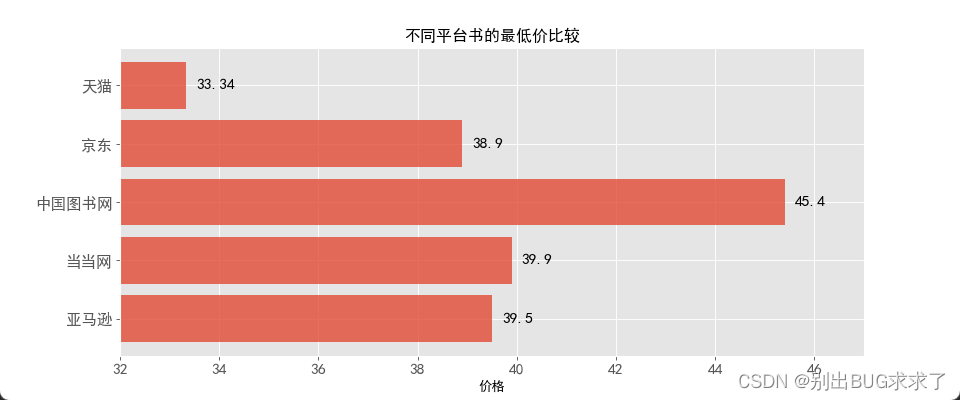代码解读
水平条形图的绘制与垂直条形图的绘制步骤一致，只是调用了barh函数来完成。需要注意的是，条形图的数值标签设置有一些不一样，需要将标签垂直居中显示，使用va参数即可。

## 2. 案例二：胡润财富榜：亿万资产超高净值家庭数

利用水平交错条形图对比2016年和2017年亿万资产超高净值家庭数（top5），其数据如下：

import matplotlib.pyplot as plt
import numpy as np
# 正常显示中文标签
plt.rcParams['font.sans-serif']=['SimHei']
# 用来正常显示负号
plt.rcParams['axes.unicode_minus']=False

plt.rcParams['axes.labelsize']=16
plt.rcParams['xtick.labelsize']=14
plt.rcParams['ytick.labelsize']=14
plt.rcParams['legend.fontsize']=16
plt.rcParams['figure.figsize']=[12,10]
plt.style.use("ggplot")
# 构建数据
Y2016 = [15600,12700,11300,4270,3620]
Y2017 = [17400,14800,12000,5200,4020]
labels = ['北京','上海','香港','深圳','广州']
bar_width = 0.35
x = np.arange(len(Y2016))
fig = plt.figure()

# 绘图
ax.bar(x,Y2016,label='Y2016',width=bar_width)
ax.bar(x+bar_width,Y2017,label='Y2017',width=bar_width)
# 添加轴标签
ax.set_xlabel('Top5城市')
ax.set_ylabel('家庭数量')
# 添加标题
ax.set_title('亿万财富家庭数Top5城市分布',fontsize=16)
# 添加刻度标签
plt.xticks(x+bar_width,labels)
# 设置Y轴的刻度范围
plt.ylim([2500, 19000])

# 为每个条形图添加数值标签
for x2016,y2016 in enumerate(Y2016):
plt.text(x2016, y2016+200, y2016,ha='center',fontsize=16)

for x2017,y2017 in enumerate(Y2017):
plt.text(x2017+0.35,y2017+200,y2017,ha='center',fontsize=16)

# 显示图例
ax.legend()
# 显示图形
plt.show()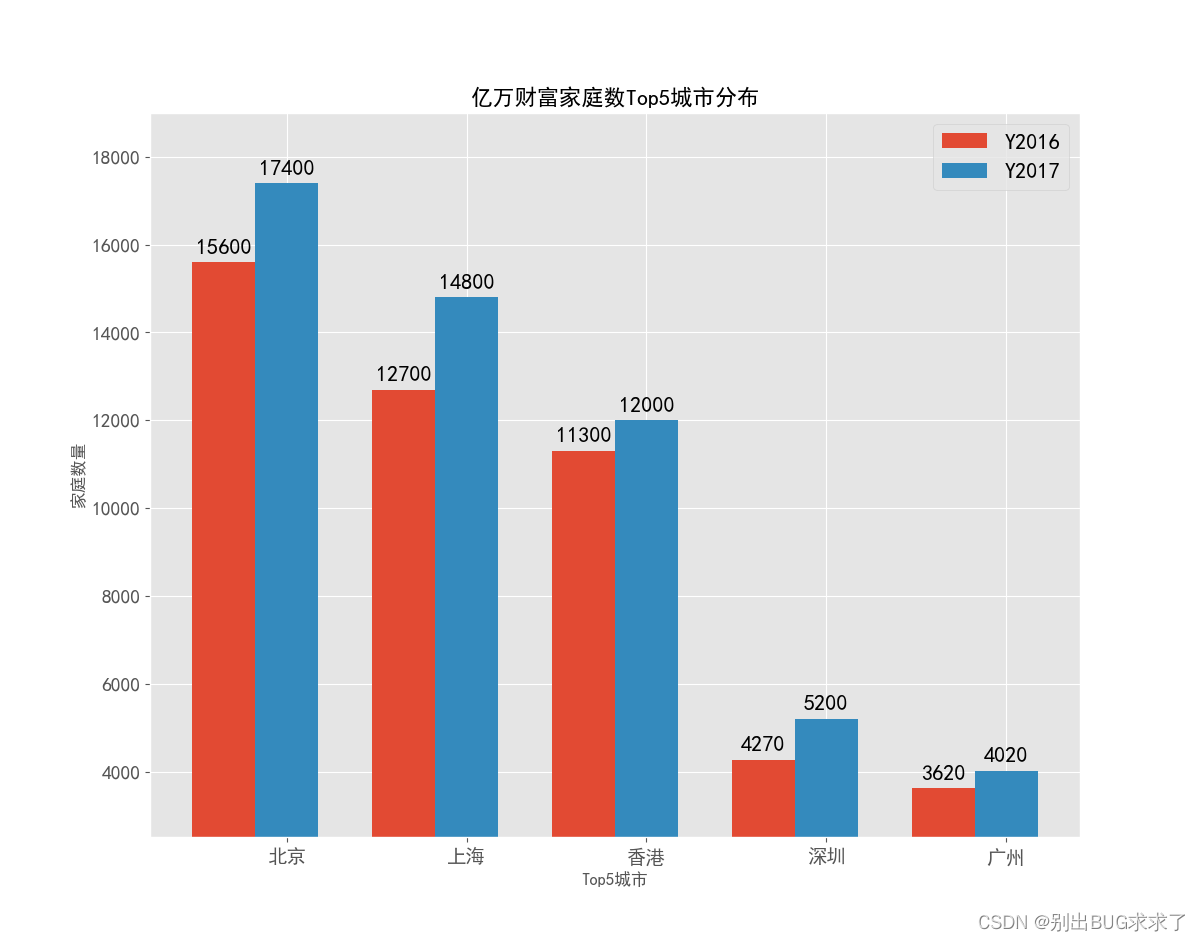代码解读

• 水平交错条形图绘制的思想很简单，就是在第一个条形图绘制好的基础上，往左移一定的距离，再去绘制第二个条形图，所以在代码中会出现两个bar函数；
• 图例的绘制需要在bar函数中添加label参数；color和alpha参数分别代表条形图的填充色和透明度；
• 给条形图添加数值标签，同样需要使用两次for循环的方式实现；
展开全文python 数据挖掘 matplotlib
• ## matplotlib画柱状图

千次阅读 2022-04-18 09:52:38
柱状图bar 柱状图（Bar Chart）是一种以长方形的长度为变量的表达图形的统计报告图，是由一系列高度不等的纵向条纹表示数据分布的情况，用来比较两个或两个以上的价值（不同时间或者不同条件），只有一个变量，通常...

柱状图bar

柱状图（Bar Chart）是一种以长方形的长度为变量的表达图形的统计报告图，是由一系列高度不等的纵向条纹表示数据分布的情况，用来比较两个或两个以上的价值（不同时间或者不同条件），只有一个变量，通常适用于较小的数据集分析。柱状图亦可横向排列，或用多维方式表达。

# 1. 主要函数

## 1.1. bar

pyplot中绘制柱状图的函数为bar，其语法格式如下。

matplotlib.pyplot.bar(x,height,*,align='center',**kwargs)

matplotlib.pyplot.bar(x,height,width,*,align='center',**kwargs)

matplotlib.pyplot.bar(x,height,width,bottom,*,align='center',**kwargs)

函数中的主要参数说明如下。

*■ x：接收array。表示x轴的数据，无默认。

*■ height：接收array。表示x轴所代表数据的数量，无默认。

■ width：接收0~1之间的float，指定柱状图宽度，默认为0.8。

■ bottom：接收array，可选，柱状图的y坐标，默认值为0。

■ align：取值为center或edge，可选，默认为center，表示柱状图对齐x坐标。

■ color：接收特定string或者包含颜色字符串的array，表示柱状图颜色，默认为None。

■ edgecolor：接收特定string或者包含颜色字符串的array，表示柱状图的边框颜色。

*■ tick_label：柱状图的刻度标签

更多参数见下图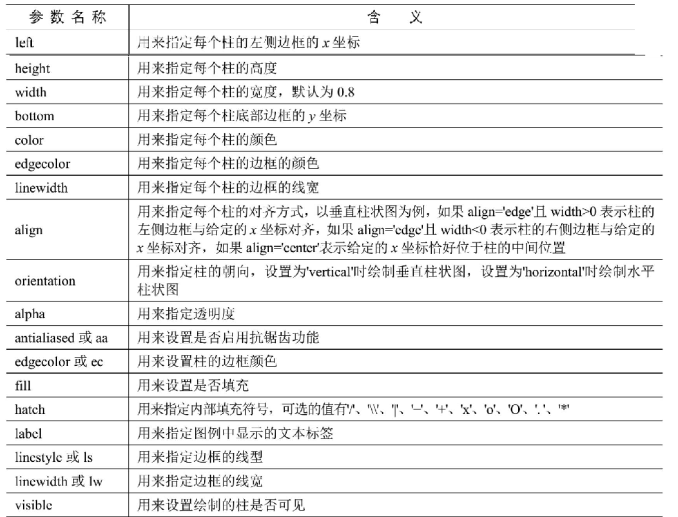# 2. 例子

## 2.1. 标准例子

import numpy as np
import matplotlib.pyplot as plt
#导入数据
Emp_data= np.loadtxt('d:\data\Employedpopulation.csv',delimiter = ",",
usecols=(1,2,3,4,5,6,7,8,9,10),dtype=int)

# 设置Matplotlib正常显示中文和负号
plt.rcParams['font.sans-serif']=['SimHei']   # 用黑体显示中文
plt.rcParams['axes.unicode_minus']=False     # 正常显示负号

#创建一个绘图对象, 并设置对象的宽度和高度
plt.figure(figsize=(6, 4))
#绘制全部就业人员柱状图
plt.bar(Emp_data,Emp_data,width = 0.35,color = 'red',
edgecolor = 'white')
#绘制城镇就业人员柱状图
plt.bar(Emp_data+0.35,Emp_data,width = 0.35,color = 'green',
edgecolor = 'white')
#绘制乡村就业人员柱状图
plt.bar(Emp_data+0.7,Emp_data, width = 0.35,color = 'blue',
edgecolor = 'white')
# 给图加text
X = Emp_data;Y1 = Emp_data
for x, y in zip(X, Y1):
plt.text(x + 0.3, y + 0.05, '%i' % y, ha='center')
Y2 = Emp_data
for x, y in zip(X, Y2):
plt.text(x + 0.6, y + 0.05, '%i' % y, ha='center')
Y3 = Emp_data
for x, y in zip(X, Y3):
plt.text(x + 0.9, y + 0.05, '%i' % y, ha='center')
#添加标签
plt.xlabel('年份')
plt.ylabel('人员(万人)')
plt.ylim((30000,80000))
plt.xlim(2006,2017)
plt.title("2007-2016年城镇、乡村和全部就业人员情况柱状图")
#添加图例
plt.legend({'全部就业','城镇就业','乡村就业'})
plt.savefig('d:/data/Employedpopulation_bar.png')
plt.show()

## 2.2. 简洁例子

import matplotlib.pyplot as plt
import numpy as np
import matplotlib.pyplot as plt

# 设置Matplotlib正常显示中文和负号
plt.rcParams['font.sans-serif']=['SimHei']   # 用黑体显示中文
plt.rcParams['axes.unicode_minus']=False     # 正常显示负号

num_list = [1.5,0.6,7.8,6]
plt.bar(range(len(num_list)), num_list,color=['r','b','g','y'])
plt.show()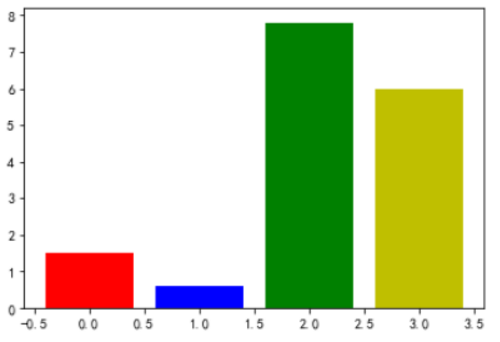## 2.3. 2个bar左右排列

import matplotlib.pyplot as plt

name_list = ['Monday','Tuesday','Friday','Sunday']
num_list = [1.5,0.6,7.8,6]
num_list1 = [1,2,3,1]
x =list(range(len(num_list)))
total_width, n = 0.8, 2
width = total_width / n

plt.bar(x, num_list, width=width, label='boy',fc = 'y')
for i in range(len(x)):
x[i] = x[i] + width
plt.bar(x, num_list1, width=width, label='girl',tick_label = name_list,fc = 'r')
plt.legend()
plt.show()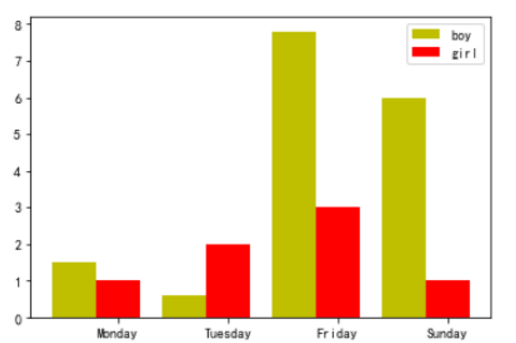## 2.4. 2个bar上下排列

import numpy as np
import matplotlib.pyplot as plt

n = 12
X = np.arange(n)
Y1 = (1-X/float(n)) * np.random.uniform(0.5,1.0,n)
Y2 = (1-X/float(n)) * np.random.uniform(0.5,1.0,n)

plt.axes([0.025,0.025,0.95,0.95])
plt.bar(X, +Y1, facecolor='#9999ff', edgecolor='white')
plt.bar(X, -Y2, facecolor='#ff9999', edgecolor='white')

for x,y in zip(X,Y1):
plt.text(x+0.4, y+0.05, '%.2f' % y, ha='center', va= 'bottom')

for x,y in zip(X,Y2):
plt.text(x+0.4, -y-0.05, '%.2f' % y, ha='center', va= 'top')

plt.xlim(-.5,n), plt.xticks([])
plt.ylim(-1.25,+1.25), plt.yticks([])
plt.show()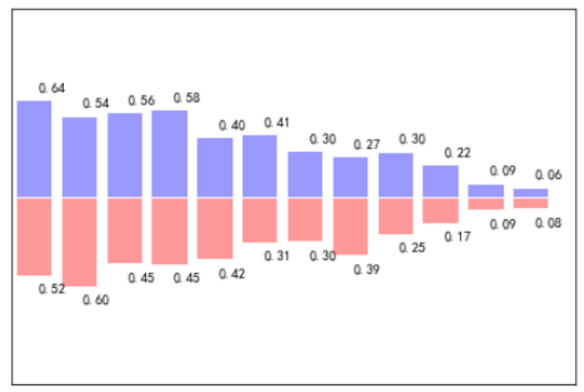## 2.5. 2个bar上下

import matplotlib.pyplot as plt
import numpy as np

n = 12
X = np.arange(n)
Y1 = (1-X/(float(n))*np.random.uniform(0.5,1.0,n))
Y2 = (1-X/(float(n))*np.random.uniform(0.5,1.0,n))

plt.bar(X,+Y1,facecolor='r',edgecolor='w')
plt.bar(X,-Y2,facecolor='b',edgecolor='w')

for x,y in zip(X,Y1):
plt.text(x-0.1,y+0.05,'%.2f'%y,ha='center',va='bottom')  #居中对齐
for x,y in zip(X,Y2):
plt.text(x+0.1,-y-0.05,'-%.2f'%y,ha='center',va='top')

plt.xlim((-0.5,n))
plt.ylim((-2,2))
plt.xticks(())
plt.yticks(())
plt.show()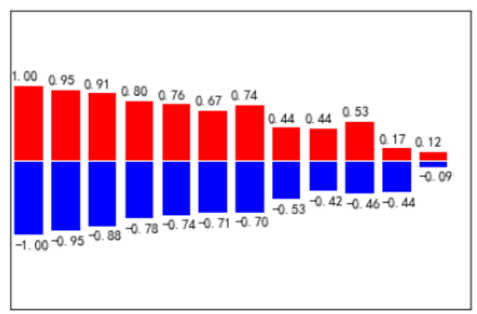展开全文python matplotlib
• 最近在总结前期的一些工作，对这些绘图工具再系统总结 matplotlib 画图汇总（柱状图python
• 1、柱状图和条形图的概念与比较 （1）标记：矩形 （2）必备的视觉通道：矩形的高（宽）度与x （y）坐标次序 常见的视觉通道：色彩、纹理、y （x）坐标绝对位置 （3）适用场景：小规模数据集中，显示各个数据大小、...python matplotlib
• 连接数据库案例 import pymysql # pip install ...import matplotlib.pyplot as plt # 用来画图（plt.plot()折线图， plt.bar()柱状图，....） plt.rcParams['font.sans-serif'] = 'SimHei' # 设置中文字体支持中matplotlib Python
• 文章目录官方示例运行...import matplotlib.pyplot as plt from matplotlib.ticker import MaxNLocator from collections import namedtuple n_groups = 5 means_men = (20, 35, 30, 35, 27) std_men = (2, 3, 4, 1python
• matplotlib是python最著名的绘图库，它提供了一整套和matlab相似的命令API，十分适合交互式地进行制图。而且也可以方便地将它作为绘图控件，嵌入GUI应用程序中。它的文档相当完备，并且Gallery页面中有上百幅缩略...
• matplotlib.pyplot 柱形添加条形颜色及宽度、设置标题文字颜色及大小1. 第一个柱形2. 垂直柱形添加中文标题3. 水平柱形添加中文标题4. 设置条形颜色宽度与标题颜色字体大小5. 四张条形对比python pycharm 机器学习
•matplotlib
• 并列柱状图的通用方法 参考链接：https://www.jb51.net/article/206226.htm datas ：数据集，二维列表，要求列表每个元素的长度必须与labels的长度一致 title：标题 x_labels : x轴坐标标签序列 y_label：y轴坐标...python matplotlib
• matplotlib 柱状图之渐变色设置 matplotlib中，在用bar或者barh绘制柱状图时，发现加入cmap是不管用的，不支持这个关键字，而且网上找了许久，也没有发现有类似功能，因此，干脆自己写一个试试，说来就来！！ ...
• 出来的效果就是题图这样，基本可以满足柱状图的绘图要求。完整代码及注释如下：import numpy as npimport matplotlib.pyplot as plt#解决显示不出中文的问题from pylab import *mpl.rcParams['font.sans-serif'] = ...
• ## 超详细的Python matplotlib 绘制柱状图

万次阅读 多人点赞 2021-11-18 11:27:14
Python 为数据展示提供了大量优秀的功能包，其中 matplotlib 模块可以方便绘制制作折线图、柱状图、散点图等高质量的数据包。 关于 matplotlib 模块，我们前期已经对matplotlib进行基本框架、以及常用方法的学习 ...python
• matplotlib没有画多列柱状图的代码，也没有人写函数，于是自己写了一个 使用时，将x_labels替换为横坐标的刻度，y为嵌套多个小列表的大列表，y中每个列表代表一个系列柱状图 其中gap必须为整数 其余参数含义可见代码...python matplotlib
• Matplotlib是一个基于python的2D画图库，能够用python脚本方便的画出折线，直方，功率谱，散点等常用图表，而且语法简单。Python中通过matplotlib模块的pyplot子库来完成绘图。Matplotlib可用于创建高质量的...
• python使用matplotlib绘制柱状图 Python绘图需要下载安装matplotlib模块，它是一个数学绘图库，我们将使用它来制作简单的图表。绘制柱状图python
• ## matplotlib绘制柱状图(基础操作)

万次阅读 多人点赞 2021-09-01 12:24:19
Matplotlib 是 Python 的绘图库。 它可与 NumPy 一起使用，提供了一种有效的 MatLab 开源替代方案。 它也可以和图形工具包一起使用，如 PyQt 和 wxPython。本文由浅入深，教你画柱状图python pycharm matplotlib
• 文章目录1、导入包2、一列柱状图3、多列柱状图 1、导入包 import warnings warnings.filterwarnings('ignore') import numpy as np import matplotlib.pyplot as plt from matplotlib import rcParams # 设置全局...python
• 柱状图(bar)1 柱状图绘制需求1-对比每部电影的票房收入电影数据如下图所示：1 准备数据['雷神3：诸神黄昏','正义联盟','东方快车谋杀案','寻梦环游记','全球风暴', '降魔传','追捕','七十七天','密战','狂兽','其它'...
• Python 中有两个专用于可视化的库，matplotlib 和 seaborn ，本文将介绍matplotlibMatplotlib：基于Python的绘图库，提供完全的 2D 支持和部分 3D 图像支持。Seaborn：该 Python 库能够创建富含信息量和美观的统计...python 画虚线
• max = sorted(domain_in)[-1:] + 100print(len(domain_name), len(domain_in)) # 生成list[0,1,2,3,4.....]x = list(range(len(domain_in))) b_list = list(range(len(median_list))) # 生成柱状图宽度total_...
• 本文代码运行在jupyter上 一.安装matplotlib pip3 install matplotlib 二.进入matplotlib查看源码 渐变柱状图官方示例python matplotlib
• 在绘制柱状图时，有时我们需要在柱体最上方显示数据 代码部分 基于python3.6 Jupyter Nootbook import matplotlib.pyplot as plt plt.rcParams['font.sans-serif'] = ['SimHei'] # 显示中文 fig = plt.figure...python
• 文章就讲解第一种图形，柱状图。1 基础绘制柱状图，我们主要用到bar()函数。只要将该函数理解透彻，我们就能绘制各种类型的柱状图。我们先看下bar()的构造函数：bar(x，height， width，*，align='center'，**kwargs...
• 柱状图 import pandas as pd import matplotlib.pyplot as plt # 遇到数据中有中文的时候，一定要先设置中文字体 plt.rcParams['font.sans-serif']=['SimHei'] # 用黑体显示中文 # 解决坐标轴负号问题 plt.rcParams...python
• 柱状图 plt.bar(x, height, color,edgecolor,width=0.8,align='center',bottom=None) 必选参数： x，表示横坐标刻度值，可以是array、list等 height，表示每个柱子的高度，可以是array、list等 可选参数： color...python
• 柱状图：排列在工作表的列或行中的数据可以绘制到柱状图中。 ...width : 柱状图宽度 align : 每个柱状图的位置对齐方式 {‘center’, ‘edge’}, optional, default: ‘center’ **kwargs : cpython...